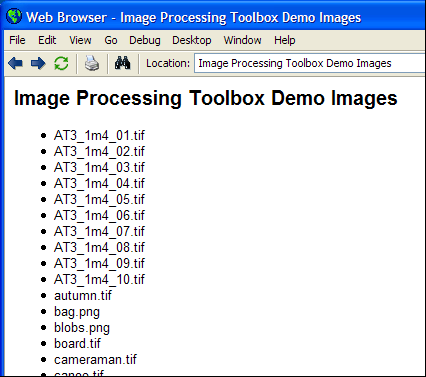# Batch processing revisited

Here's a very common question we receive:

"How do I process a set of image files in a directory?"

I first posted an example illustrating "batch processing" of a set of image files a couple of years ago. The techniques are not specific to image processing, though. Loren covered this topic in her Art of MATLAB blog, and Doug posted a tutorial video recently in Pick of the Week.

Today I've got a new batch processing example for you. It was inspired by this question someone asked me recently: How can I get a list of all the sample images shipped with the Image Processing Toolbox? This question provides a good excuse to show a variety of different MATLAB tools and techniques that you might not have seen before, including:

• Functions for manipulating file paths in a platform-independent manner
• Determining all the file formats supported by imread and imwrite, as well as the recognized file extensions for each format.
• Using the comma-separated list syntax on structure arrays
• Sorting a structure array according to one of its fields
• Generating HTML dynamically and displaying it in the MATLAB Web Browser.

All of the toolbox sample images are stored in the same subdirectory of the MATLAB installation directory. We can use matlabroot and fullfile to find the complete path.

matlabroot
ans =

C:\Program Files\MATLAB\R2008a


demos_folder = fullfile(matlabroot, 'toolbox', 'images', 'imdemos')
demos_folder =

C:\Program Files\MATLAB\R2008a\toolbox\images\imdemos



The purpose of fullfile is to produce a valid path string on all MATLAB platforms. That way, you don't have to worry about whether your code will be running on a platform that uses "/" or "\" as the path separator.

Did you know you can automatically determine all of the file formats supported by imread and imwrite? The secret is to use a little-known function called imformats. This function returns a struct array containing information about format descriptions, recognized file extensions, and functions to be used for reading and writing each format.

f = imformats
f =

1x16 struct array with fields:
ext
isa
info
read
write
alpha
description



The number of elements of the structure array is the number of recognized image file formats.

num_formats = numel(f)
num_formats =

16



Each element of the structure contains information about a particular format. This information is used by imread, imwrite, and imfinfo.

f(1)
ans =

ext: {'bmp'}
isa: @isbmp
info: @imbmpinfo
read: @readbmp
write: @writebmp
alpha: 0
description: 'Windows Bitmap (BMP)'



The ext field of the struct array is a cell array containing the recognized filename extensions for each format.

f(1).ext
ans =

'bmp'



The reason ext is a cell array is that multiple extensions are recognized for certain formats. For example:

f(3).ext
ans =

'fts'    'fits'


f(7).ext
ans =

'jpg'    'jpeg'



Use the comma-separated list syntax to create a single list of all recognized extensions.

extensions = [f.ext]
extensions =

Columns 1 through 7

'bmp'    'cur'    'fts'    'fits'    'gif'    'hdf'    'ico'

Columns 8 through 14

'jpg'    'jpeg'    'pbm'    'pcx'    'pgm'    'png'    'pnm'

Columns 15 through 19

'ppm'    'ras'    'tif'    'tiff'    'xwd'



Now build up a list of image files in the Image Processing Toolbox demos folder. Use dir in a loop to do directory listings of *.jpg, *.tif, *.bmp, etc.

% Initialize an empty struct array.
files = struct([]);
for k = 1:numel(extensions)
files_k = dir(fullfile(demos_folder, sprintf('*.%s', extensions{k})));
files = [files; files_k];
end

files
files =

64x1 struct array with fields:
name
date
bytes
isdir
datenum


files(1)
ans =

name: 'solarspectra.fts'
date: '13-Oct-2002 08:48:02'
bytes: 66240
isdir: 0
datenum: 7.3150e+005


files(2)
ans =

name: 'football.jpg'
date: '01-Mar-2001 09:52:38'
bytes: 27130
isdir: 0
datenum: 7.3091e+005



We might want to sort the image files alphabetically. Do this by:

1. Make a cell array of file names.

2. Sort the cell array of file names and save the second output from sort. (The second output is called the permutation vector of the sort.)

3. Rearrange the structure array using the permutation vector.

Step 1:

filenames = {files.name};

Step 2:

[filenames_sorted, idx] = sort(filenames);

Step 3:

files = files(idx);

Now we construct HTML code for a page that displays all the sample image files in a bulleted list.

html = cell(0,0);
html{end+1} = '<html>';
html{end+1} = '<head>';
html{end+1} = '<title>Image Processing Toolbox Demo Images</title>';
html{end+1} = '</head>';
html{end+1} = '<body>';
html{end+1} = '<h2>Image Processing Toolbox Demo Images</h2>';
html{end+1} = '<ul>';

for k = 1:numel(files)
html{end+1} = '<li>';
html{end+1} = files(k).name;
html{end+1} = '</li>';
end

html{end+1} = '</ul>';
html{end+1} = '</body>';
html{end+1} = '</html>';

We could save the generated HTML into a file. But we can also display the HTML directly in the MATLAB Web Browser without saving it. To do that, we need to:

1. Construct a single character array that contains all of the HTML.

2. Prepend 'text://' to the HTML code.

3. Pass the resulting string to the web function.

html_str = sprintf('%s\n', html{:});
web(['text://', html_str])

Here's a screen shot showing the results in the MATLAB Web Browser:Published with MATLAB® 7.6

|

### Comments

To leave a comment, please click here to sign in to your MathWorks Account or create a new one.